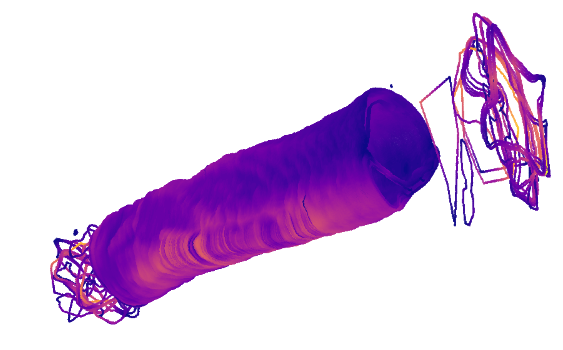# Color Surface with thinkness values

Hi,
I’m trying to color a surface generated from vtkImageData using the values from another vtkImageData. This is the code to generate the surface:

``````surface = vtk.vtkMarchingCubes()
surface.SetInputData(imgstenc.GetOutput())
surface.ComputeNormalsOn()
surface.SetValue(0, 127.5)
``````

Where “imgstenc” is an vtkImageStencil object that generates a binary image from a bone surface mesh. Then I process the binary image to compute the thickness of the bone. Results are in another vtkImageData variable called “thickness” with the same dimensions, origin and spacing than “imgstenc”.

I’m trying to color each cell from the surface object using the values from the “thickness” vtkImageData to obtain something like this:Where warmer colors mean larger values of thickness. I’ve been trying to assign the thickness value to each surface cell with something like this:

``````color = vtk.vtkUnsignedCharArray()
color.SetNumberOfComponents(1)
color.SetNumberOfTuples(  surface.GetOutput().GetNumberOfCells() )
for i in range(surface.GetOutput().GetNumberOfCells()):
color.InsertTuple(i, <????>)
``````

But I don’t know how to extract the corresponding thickness value to each surface cell. Any idea??

Thanks a lot!

I finaly sorted this out. Those are the steps.

1. Create surface from the vtkImageStencil object:
``````surface = vtk.vtkMarchingCubes()
surface.SetInputData(imgstenc.GetOutput())
surface.ComputeNormalsOn()
surface.SetValue(0, 127.5)
``````
1. Create a ProbeFilter sampling your vtkImageData with the thickness values with the points of your PolyData surface mesh:
``````probe = vtk.vtkProbeFilter()
probe.SetInputData(surface.GetOutput())
probe.SetSourceData(thickness)
probe.Update()
``````
1. Plot the sampled points with the thickness values:
``````rng = thickness.GetScalarRange()
fMin = rng
fMax = rng
print("RANGE:", rng,rng)

# Make the lookup table.
lut = vtk.vtkLookupTable()
lut.SetTableRange(fMin, fMax)
lut.SetSaturationRange(0.5, 1)
lut.SetHueRange(0.7, 0.9)
lut.SetValueRange(0, 5)
lut.Build()

normals = vtk.vtkPolyDataNormals()
normals.SetInputConnection(probe.GetOutputPort())

mapper = vtk.vtkPolyDataMapper()
mapper.ScalarVisibilityOn()
mapper.SetLookupTable(lut)
mapper.SetInputConnection(normals.GetOutputPort())
mapper.SetScalarRange(fMin, fMax)

actor = vtk.vtkActor()
actor.SetMapper(mapper)
``````

That worked for me.